# PB'05: solvers page (graphs)

List of figures

## Number of instances that can be solved in a fixed amount of time (by category)

These graphs represent the number of answers (x axis) that a solver is able to provide within a given time limit (y axis). They are generated automatically. Some of them are not meaningful because there are too few points. However, they are given here for completeness.

### Category "no optimization function" (SAT)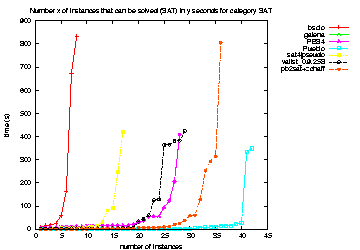SAT answers for category "no optimization function" (SAT)(PDF format here)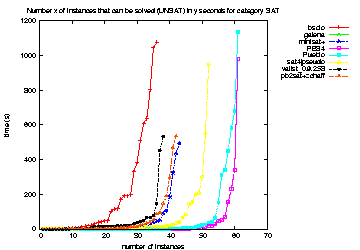UNSAT answers for category "no optimization function" (SAT)(PDF format here)

### Category "optimization, small integers" (OPTSMALLINT)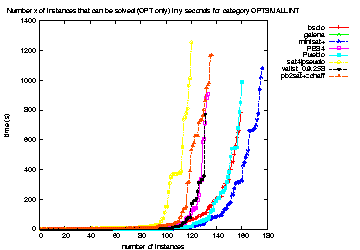OPTIMUM FOUND answers for category "optimization, small integers" (OPTSMALLINT)(PDF format here)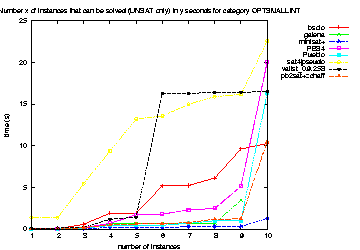UNSAT answers for category "optimization, small integers" (OPTSMALLINT)(PDF format here)

### Category "optimization, medium integers" (OPTMEDINT)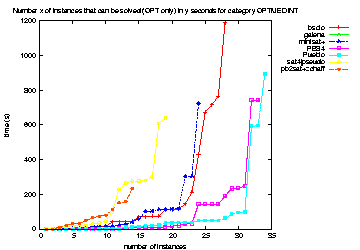OPTIMUM FOUND answers for category "optimization, medium integers" (OPTMEDINT)(PDF format here)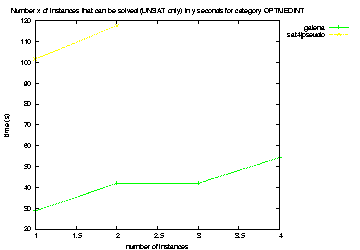UNSAT answers for category "optimization, medium integers" (OPTMEDINT)(PDF format here)

### Category "optimization, big integers" (OPTBIGINT)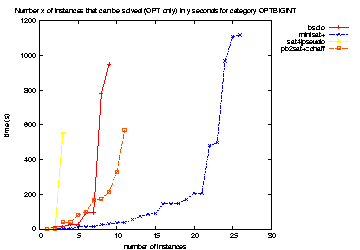OPTIMUM FOUND answers for category "optimization, big integers" (OPTBIGINT)(PDF format here)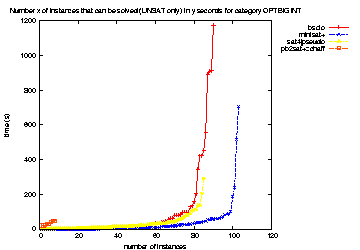UNSAT answers for category "optimization, big integers" (OPTBIGINT)(PDF format here)

### All categories

The graphs below represent the OPTIMUM FOUND and UNSAT answers for all categories. Remember however that some solvers had no support for some categories (especially big integers). Even if it makes sense to consider that a solver which has no support for a category gives no answer in that category, the curves should be compared with some care.
Here are the categories which were supported by each solver

SolverSATOPTSMALLINTOPTMEDINTOPTBIGINT
bsolo
galena
minisat+
PBS4
Pueblo
sat4jpseudo
vallst_0.9.258
pb2sat+zchaff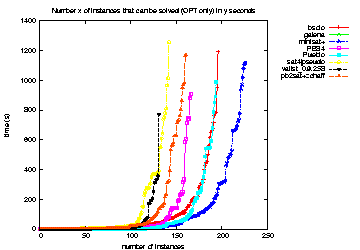OPTIMUM FOUND answers for all categories(PDF format here)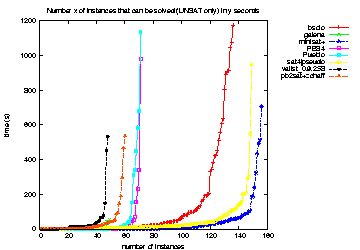UNSAT answers for all categories(PDF format here)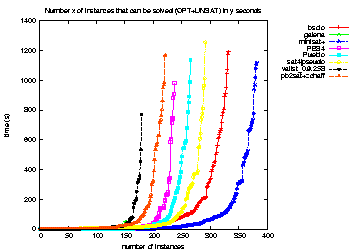OPTIMUM FOUND and UNSAT answers for all categories(PDF format here)